# Holidays with grandmam

We have packed three T-shirts - white, red, orange and five pants - blue, green, black, pink and yellow. How many days can we spend with the old mother if we put on a different combination of clothes every day?

Result

t =  15

#### Solution:Leave us a comment of example and its solution (i.e. if it is still somewhat unclear...):Be the first to comment!#### To solve this example are needed these knowledge from mathematics:

Would you like to compute count of combinations?

## Next similar examples:

1. Families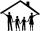In class we investigated how many children have family in which its live. How many% of pupils live in families with more than two children? If five families each have one child in 9 families have 2 children, in 5 families have 3 children, in 2 families ha
2. Street numbersLada came to aunt. On the way he noticed that the houses on the left side of the street have odd numbers on the right side and even numbers. The street where he lives aunt, there are 5 houses with an even number, which contains at least one digit number 6.
3. Five-digitFind all five-digit numbers that can be created from numbers 12345 so that the numbers are not repeated and then numbers with repeated digits. Give the calculation.
4. Florist's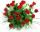The florist got 72 white and 90 red roses. How many bouquets can bind from all these roses when each bouquets should have the same number of white and red roses?
5. Three digits number 2Find the number of all three-digit positive integers that can be put together from digits 1,2,3,4 and which are subject to the same time has the following conditions: on one positions is one of the numbers 1,3,4, on the place of hundreds 4 or 2.
6. SickSick Marcel already taken six tablets, which was a quarter of the total number of pills in the pack. How many pills were in the pack?
7. The largest numberFind the largest integer such that: 1. No figures is not repeat, 2. multiplication of every two digits is odd, 3. addition all digits is odd.
8. SymbolsIf 2*3 = 60 ; 3*4 = 120 and 4*5 = 200, what is 2*5?
9. Simplify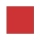Simplify powers multiplication: (3+22)(5-42)
10. Crocuses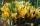The garden grow daffodils, crocuses and roses. 1400 daffodils, crocuses is 462 more and roses is 156 more than crocuses. How many are all the flowers in the garden?
11. MidpointsTriangle whose sides are midpoints of sides of triangle ABC has a perimeter 45. How long is perimeter of triangle ABC?
12. InfirmaryTwo thirds of children from the infirmary went on a trip seventh went to bathe and 40 children remained in the gym. How many children were treated in the infirmary?
13. Tickets 3A total of 645 tickets were sold for the school play. They were either adult tickets or student tickets. There were 55 fewer student tickets sold than adult tickets. How many adult tickets were sold?
14. Pins 2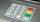how many different possible 4 digits pins can be found on the 10-digit keypad?
15. Average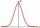Average of 7 numbers is 65. What is its sum?
16. Simple equationSolve the following simple equation: 2. (4x + 3) = 2-5. (1-x)
17. Write 2Write 791 thousandths as fraction in expanded form.